# Excel String Functions – LEFT, RIGHT, MID, LEN, and FIND

• Last Updated : 21 Aug, 2021

Excel is mostly about numerical, however, we often have data of text data type. Here are a few functions we should know to handle text data.

• LEFT
• RIGHT
• MID
• LEN
• FIND

## The LEFT function

The LEFT function returns a given text from the left of our text string based on the number of characters specified.

Attention reader! Don’t stop learning now. If you are an Excel beginner (or an intermediate) and want to learn Excel, Geeksforgeeks brings the perfect course for you to start, Diving Into Excel

Syntax:

`LEFT(text, [num_chars])`

Parameters:

• Text: The text we want to extract from.
• Num_chars (Optional): The number of characters you want to extract. Default num_chars is 1 and the number must be a positive number that is greater than zero.

Example:

Now if you want to get the first “Geeks” from “Geeksforgeeks” in B2. Let us follow the next step.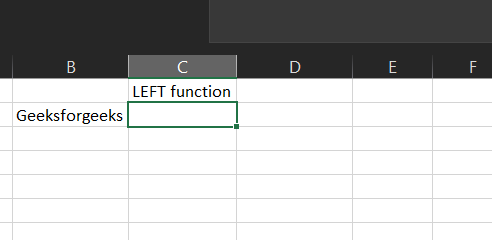Step 2: We will enter =LEFT(B2,5) in the B3 cell. Here we want Excel to extract the first 5 characters from the left side of our text in B2.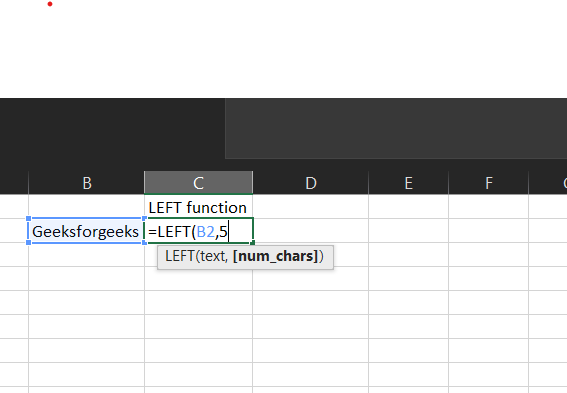This will return “Geeks”.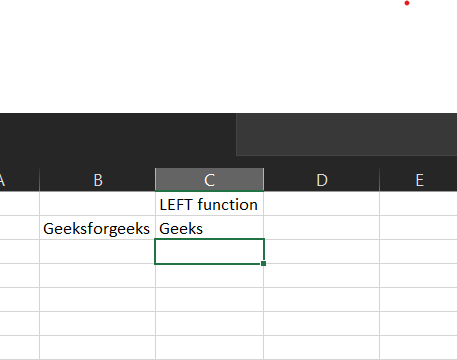## The RIGHT Function

The RIGHT function returns a given text from the left of our text string based on the number of characters specified.

Syntax:

`RIGHT(text, [num_chars])`

Parameters:

• Text: The text we want to extract from.
• Num_chars (Optional): The number of characters you want to extract. Default num_chars is 1 and the number must be a positive number that is greater than zero.

Example:

Now if you want to get the last geeks from “Geeksforgeeks” in B2. Let us follow the next step.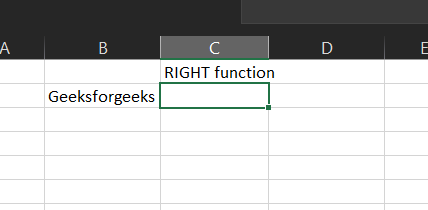Step 2: We will enter =RIGHT(B2,5) in the B3 cell. Here we want Excel to extract the last 5 characters from the right side of our text in B2.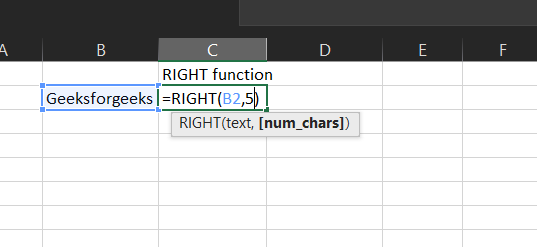This will return “geeks”.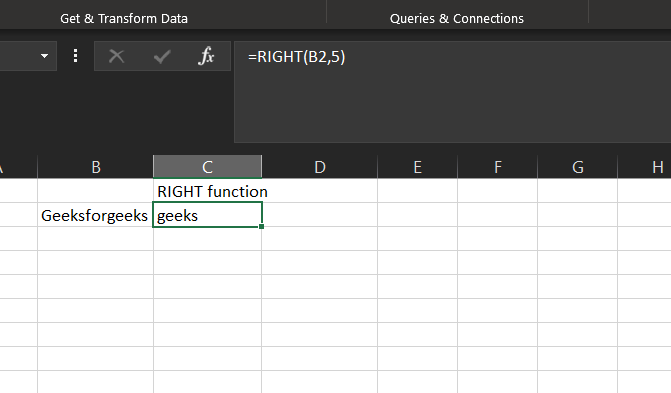## The MID function

The MID function returns the text from any middle part of our text string based on the starting position and number of characters specified.

Syntax:

`MID(text, start_num, num_chars)`

Parameters:

• Text: The text we want to extract from.
• start_num: The starting number of the first character from the text we want to extract.
• Num_chars: The number of characters you want to extract.

Example: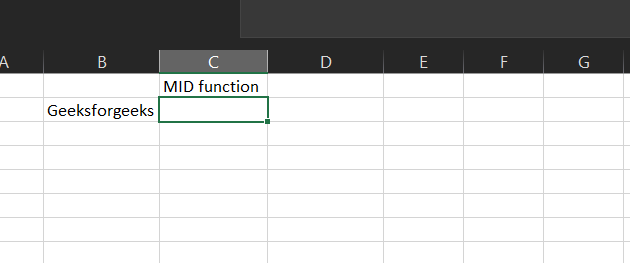Now if you want to get the character “for” which is located in the middle of our text “Geeksforgeeks” in B2. Let us follow the next step.

Step 2: We will enter =MID(B2,5,3) in the B3 cell. Here we want Excel to extract the characters located in the middle of our text in B2.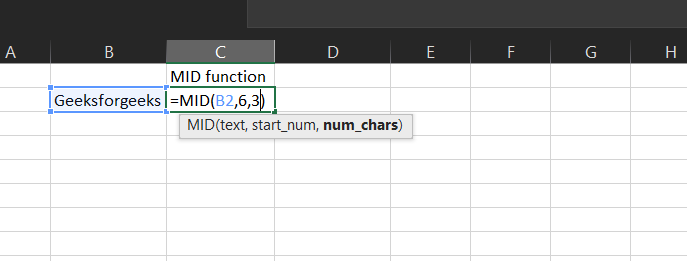This will return “for”.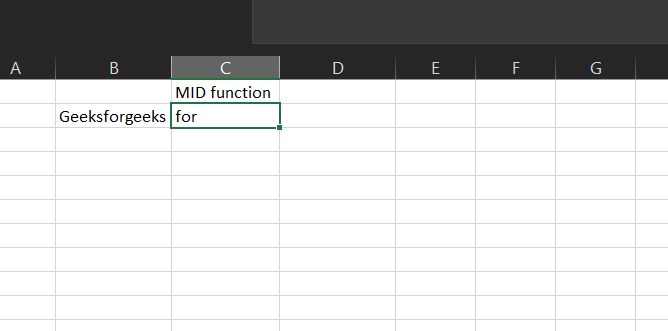## The Len Function

The LEN function returns the number of characters in the text strings.

Syntax:

`LEN(text)`

Parameters:

• Text: The text we want to know the length

Example:

Now if you want to know how many characters are in the text “Geeksforgeeks”. Let us follow the next step.Step 2: We will enter =LEN(B2) in the B3 cell. Excel will count how many characters are in the text.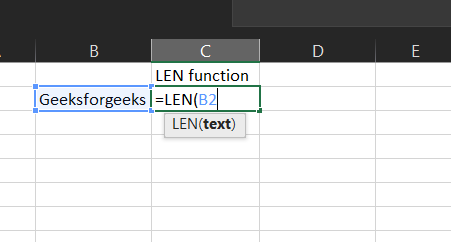This will return 13.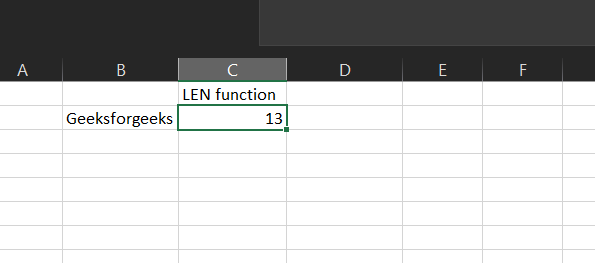## The FIND Function

The FIND function returns the position of a given text within a text.

Syntax:

`FIND(find_text, within_text, [start_num])`

Parameters:

• Find_text: The text we want to find.
• Within_text: The text containing our find_text.
• Start_num (Optional): The starting position of our find_text. Default is 1.

Example: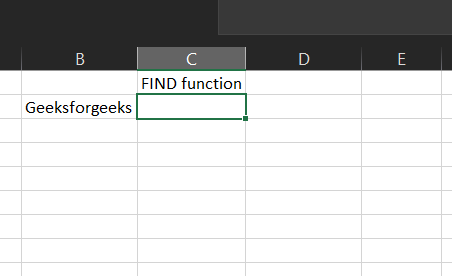Now if you want to find “for” in geeksforgeeks in B2. Let us follow the next step.

Step 2: We will enter =FIND(“for”,B2) in B3 cell. Here we want Excel to find “for” in our text in B2.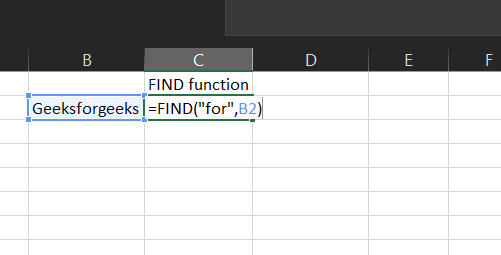This will return 6 because “for” is located at character number 6 in our text.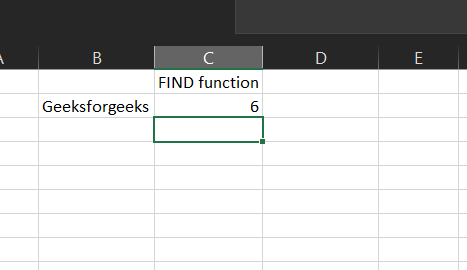My Personal Notes arrow_drop_up